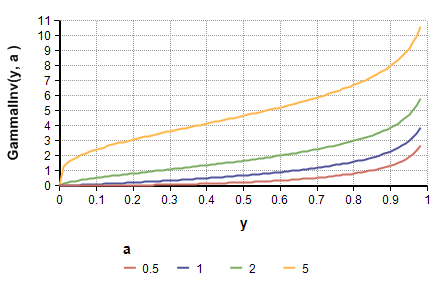# GammaIInv

## GammaIInv(y, a, b)

Returns the inverse of the incomplete Gamma function. The function returns the value x such that

GammaI(x, a, b) = y

«a» is the shape parameter, «b» is an optional scale factor that defaults to 1.

GammaIInv(Uniform(0, 1), a, b) is equivalent to Gamma(a, b).

GetFract(Gamma(a, b), y) is an approximation to GammaIInv(y, a, b), obtained via sampling. The former is subject to sampling error, with less error at increased sample sizes, whereas by using GammaIInv directly, sampling error is avoided entirely.

## Library

Advanced Math, Distribution Densities Library (Distribution Densities.ana)

## Examples

Index y := Sequence(0,0.98, 0.01)
Variable a := [0.5, 1, 2, 5]
GammaIInv(y, a) →GammaI(12, 5, 2) → 0.7149
GammaIInv(0.7149, 5, 2) → 12